# 字典和集聚，关于Python数据结构中字典的涉世

python高级——目录

### python高级系列文章目录

python高级——目录

``````>>> data = [(1, 'a'), (2, 'b'), (3, 'c')]
>>> data_dict = {num: letter for num, letter in data}
>>> data_dict
{1: 'a', 2: 'b', 3: 'c'}
``````

### 字典

 ``````''' 字典是python内置类型中唯一的映射，先看创建字典的几种方法 1、对象创建 2、大括号 3、zip ''' if __name__ == "__main__": # 1、利用实例化对象的方法创建 a = dict(key1=1, key2=2, all=[1, 2, 3]) b = dict([('key3', 3), ('key4', 4)]) c = dict({"key5": 5, "key6": 6}) print("a:", a) # a: {'key1': 1, 'all': [1, 2, 3], 'key2': 2} print("b:", b) # b: {'key3': 3, 'key4': 4} print("c:", c) # c: {'key6': 6, 'key5': 5} # 2、直接使用大括号 d = {"key7": 7, "key8": 8} print("d:", d) # d: {'key8': 8, 'key7': 7} # 3、使用zip e = dict(zip(("key9", "key10", "key11"), [9, 10, 11])) print("e:", e) # e: {'key11': 11, 'key10': 10, 'key9': 9}``````

（1）字典推导式

 ``````''' 字典推导式：字典推导式的创建方法同列表推导式类似 以下直接引用《流畅的python》中的例子 ''' if __name__ == "__main__": DIAL_CODES = [ (86, 'China'), (91, 'India'), (1, 'United States'), (62, 'Indonesia'), (55, 'Brazil'), (92, 'Pakistan'), (880, 'Bangladesh'), (234, 'Nigeria'), (7, 'Russia'), (81, 'Japan'), ] country_code = {country: code for code, country in DIAL_CODES} print(country_code) # {'Russia': 7, 'Indonesia': 62, 'Brazil': 55, 'China': 86, 'India': 91, 'Bangladesh': 880, 'Pakistan': 92, 'United States': 1, 'Nigeria': 234, 'Japan': 81} code_upper = {code: country.upper() for country, code in country_code.items() if code < 66} print(code_upper) # {1: 'UNITED STATES', 7: 'RUSSIA', 62: 'INDONESIA', 55: 'BRAZIL'}``````
``````（2）处理不存在的键
``````
 ``````''' 处理找不到的键 在实际场景中，当使用d[key]的方法查找数据的时候，如果找不到该键，python会抛出KeyError异常； 如果是取值操作，可以使用d.get(key, default)来解决，可以给找不到的键一个默认的值 但是如果要给更新某个不存在键对应的值的时候，就稍显麻烦了，可以使用以下方法解决： 1、用setdefault处理dict找不到的键 2、使用defaultdict对象 3、__missing__方法 ''' class Foo: def __init__(self, name=None): self.name = name def __repr__(self): return str(self.name) def setattr(self, key, value): self.__setattr__(key, value) return self if __name__ == "__main__": d1 = {} print(d1.get("key", "default")) # default 使用d.get(key, default)的方法取值 # 1、用setdefault处理dict找不到的键 d2 = {} d2.setdefault("key", [x for x in "adfaf"]) # setdefault虽然是set名字，但是是取值操作，只有当键不存在时才进行赋值，并返回该值 l = d2.setdefault("key", []) print(l) # ['a', 'd', 'f', 'a', 'f'] d2.setdefault("key2", []).extend([1, 2, 3]) # 返回空列表，所以可在后面直接使用方法extend print(d2) # {'key': 'default', 'key2': [1, 2, 3]} # 2、使用defaultdict对象 # 在python中，还有一些dict的变种类型，defaultdict为其中一种，位于collections中 from collections import defaultdict dic = defaultdict(list) # 将list的构造方法作为default_factory（只有__getitem__找不到值时调用） dic["key"].extend([1, 2, 3]) # dic中不含有"key"键，此时default_factory会被调用，创造一个空列表,并连接[1, 2, 3] print(dic["key"]) # [1, 2, 3] dic = defaultdict(Foo) # 将Foo的构造方法作为default_factory创建一个defaultdict print(dic["key"].setattr("name", "default")) # default # 3、__missing__方法 # 所有的映射类型在找不到键的时候，都会牵扯到__missing__方法；如果在__getitem__找不到键的时候，python就会自动调用它 # 另外，__missing__方法只会被getitem调用，对get或者__contains__没有影响 class My_dict(dict): def __missing__(self, key): print("正在调用__missing__...") mdict = My_dict(one=1, two=2, three=3) print(mdict) # {'two': 2, 'three': 3, 'one': 1} mdict["key"] # 正在调用__missing__...``````
``````（3）字典的变种
``````
 ``````''' 在python中虽然只有dict为映射类型，但是dict有很多变种，上面defaultdict就是，除此之外还有： （1）OrderedDict: 有顺序的字典 (2) ChainMap： 可以容纳数个不同的映射对象 (3) Counter： 给键准备一个整数计数器，每次更新键的时候会增加该计数器 （4）UserDict： 将标准的dict用python实现了一遍 ''' from collections import OrderedDict, ChainMap, Counter, UserDict if __name__ == "__main__": # 1、OrderedDict d = OrderedDict() d['one'] = 1 d['two'] = 2 d['three'] = 3 for _ in range(10): print("%d次：" % _) for k, v in d.items(): print("**", k, v) # OrderedDict迭代的时候的顺序总是跟插入顺序一致 # 2、ChainMap pylookup = ChainMap(d, globals()) # d和globals()都是映射类型，ChainMap会将其组合 for v, k in pylookup.items(): print(v, k) # 3、Counter ct = Counter('asfjlajslfjals') print(ct) # Counter({'j': 3, 'l': 3, 's': 3, 'a': 3, 'f': 2}) # 存储的是每个字母出现的次数 ct.update('jjjjjjjjlllllllll') print(ct) # # Counter({'l': 12, 'j': 11, 's': 3, 'a': 3, 'f': 2}) import random ct2 = Counter([random.randrange(1, 5) for _ in range(100)]) # 列表推导式创建Counter print(ct2) # Counter({1: 30, 2: 24, 4: 24, 3: 22}) ct3 = Counter((random.randrange(1, 5) for _ in range(100))) # 生成器创建Counter print(ct3) # Counter({2: 40, 3: 23, 4: 20, 1: 17}) class Foo: def __init__(self, num): self.l = [random.randrange(1, 5) for _ in range(num)] def __iter__(self): return iter(self.l) ct4 = Counter(Foo(100)) # 可迭代对象创建Counter print(ct4) # Counter({2: 31, 3: 25, 4: 25, 1: 19}) # 4、UserDict # 创建自定义的映射类型，一般以UserDict为基类 class My_dict(UserDict): def __missing__(self, key): if isinstance(key, str): raise KeyError(key) return self[str(key)] def __contains__(self, key): return str(key) in self.data def __setitem__(self, key, item): print("调用__setitem__。。。") self.data[str(key)] = item mdict = My_dict() mdict["one"] = 1 # 调用__setitem__。。。（下同） mdict["two"] = 2 mdict["three"] = 3 print(mdict) # {'three': 3, 'one': 1, 'two': 2}``````

### 字典

 ``````''' 字典是python内置类型中唯一的映射，先看创建字典的几种方法 1、对象创建 2、大括号 3、zip ''' if __name__ == "__main__": # 1、利用实例化对象的方法创建 a = dict(key1=1, key2=2, all=[1, 2, 3]) b = dict([('key3', 3), ('key4', 4)]) c = dict({"key5": 5, "key6": 6}) print("a:", a) # a: {'key1': 1, 'all': [1, 2, 3], 'key2': 2} print("b:", b) # b: {'key3': 3, 'key4': 4} print("c:", c) # c: {'key6': 6, 'key5': 5} # 2、直接使用大括号 d = {"key7": 7, "key8": 8} print("d:", d) # d: {'key8': 8, 'key7': 7} # 3、使用zip e = dict(zip(("key9", "key10", "key11"), [9, 10, 11])) print("e:", e) # e: {'key11': 11, 'key10': 10, 'key9': 9}``````
 ``````''' 字典推导式：字典推导式的创建方法同列表推导式类似 以下直接引用《流畅的python》中的例子 ''' if __name__ == "__main__": DIAL_CODES = [ (86, 'China'), (91, 'India'), (1, 'United States'), (62, 'Indonesia'), (55, 'Brazil'), (92, 'Pakistan'), (880, 'Bangladesh'), (234, 'Nigeria'), (7, 'Russia'), (81, 'Japan'), ] country_code = {country: code for code, country in DIAL_CODES} print(country_code) # {'Russia': 7, 'Indonesia': 62, 'Brazil': 55, 'China': 86, 'India': 91, 'Bangladesh': 880, 'Pakistan': 92, 'United States': 1, 'Nigeria': 234, 'Japan': 81} code_upper = {code: country.upper() for country, code in country_code.items() if code < 66} print(code_upper) # {1: 'UNITED STATES', 7: 'RUSSIA', 62: 'INDONESIA', 55: 'BRAZIL'}``````
 ``````''' 处理找不到的键 在实际场景中，当使用d[key]的方法查找数据的时候，如果找不到该键，python会抛出KeyError异常； 如果是取值操作，可以使用d.get(key, default)来解决，可以给找不到的键一个默认的值 但是如果要给更新某个不存在键对应的值的时候，就稍显麻烦了，可以使用以下方法解决： 1、用setdefault处理dict找不到的键 2、使用defaultdict对象 3、__missing__方法 ''' class Foo: def __init__(self, name=None): self.name = name def __repr__(self): return str(self.name) def setattr(self, key, value): self.__setattr__(key, value) return self if __name__ == "__main__": d1 = {} print(d1.get("key", "default")) # default 使用d.get(key, default)的方法取值 # 1、用setdefault处理dict找不到的键 d2 = {} d2.setdefault("key", [x for x in "adfaf"]) # setdefault虽然是set名字，但是是取值操作，只有当键不存在时才进行赋值，并返回该值 l = d2.setdefault("key", []) print(l) # ['a', 'd', 'f', 'a', 'f'] d2.setdefault("key2", []).extend([1, 2, 3]) # 返回空列表，所以可在后面直接使用方法extend print(d2) # {'key': 'default', 'key2': [1, 2, 3]} # 2、使用defaultdict对象 # 在python中，还有一些dict的变种类型，defaultdict为其中一种，位于collections中 from collections import defaultdict dic = defaultdict(list) # 将list的构造方法作为default_factory（只有__getitem__找不到值时调用） dic["key"].extend([1, 2, 3]) # dic中不含有"key"键，此时default_factory会被调用，创造一个空列表,并连接[1, 2, 3] print(dic["key"]) # [1, 2, 3] dic = defaultdict(Foo) # 将Foo的构造方法作为default_factory创建一个defaultdict print(dic["key"].setattr("name", "default")) # default # 3、__missing__方法 # 所有的映射类型在找不到键的时候，都会牵扯到__missing__方法；如果在__getitem__找不到键的时候，python就会自动调用它 # 另外，__missing__方法只会被getitem调用，对get或者__contains__没有影响 class My_dict(dict): def __missing__(self, key): print("正在调用__missing__...") mdict = My_dict(one=1, two=2, three=3) print(mdict) # {'two': 2, 'three': 3, 'one': 1} mdict["key"] # 正在调用__missing__...``````

### 泛映射类型

 ``````''' 泛映射类型就是广义上的对应关系，在数学中，我们将集合A对应集合B中的对应法则称为"映射"（Mapping） 同样，在python里，我们称"键值对"为映射，这其实也是一种对应法则 如果一个数据类型是映射，那么它肯定属于collections.abc.Mapping，可使用isinstance函数测试 PS: 字典是 Python 语言中唯一的映射类型。映射类型对象里哈希值(键) 和指向的对象(值)是一对多的关系。 ''' from collections import abc # 我们测试一些常用的类型是不是映射 if __name__ == "__main__": print(isinstance({}, abc.Mapping)) # True 字典是典型的键值对 print(isinstance([1, 2], abc.Mapping)) # False 列表是序列 print(isinstance((1, 2), abc.Mapping)) # False 元组是序列 print(isinstance('adfasfd', abc.Mapping)) # False 字符串也是序列``````
 ``````''' 大家可以查看_collections_abc.py源代码，里面基本的类型包含: ["Awaitable", "Coroutine", "AsyncIterable", "AsyncIterator", "Hashable", "Iterable", "Iterator", "Generator", "Sized", "Container", "Callable", "Set", "MutableSet", "Mapping", "MutableMapping", "MappingView", "KeysView", "ItemsView", "ValuesView", "Sequence", "MutableSequence", "ByteString", ] '''``````

 ``````''' 如果我们自己想定义一个映射类型的对象，那么必须实现__getitem__、__iter__、__len__方法 PS：关于该部分的原理，本人暂未查看说明文档，毕竟现实中几乎不可能自定义映射；有兴趣的同志可深入钻研。 ''' class Foo(abc.Mapping): def __init__(self, name): self.name = name def __getitem__(self, item): return self.name def __iter__(self): return iter(str(self.name)) def __len__(self): return len(self.name) print(isinstance(Foo("123"), abc.Mapping)) # True``````

## python高级（三）—— 字典和集合（泛映射类型），python映射

default_factory 并不是一个方法，而是一个可调用对象，它的值 defaultdict 初始化的时候由用户设定。 OrderedDict.popitem() 会移除字典最先插入的元素（先进先出）；可选参数 last 如果值为真，则会移除最后插入的元素（后进先出）。用 setdefault 处理找不到的键

### 映射的再讨论

 ``````''' python标准库里面的映射类型都是可变的，有时候需要使用不可变的映射，从python3.3开始，types模块中引入了 MappingProxyType类，如果给这个类一个映射，那么它会返回这个映射的试图，该试图是动态的，原映射如果有改动 可立即通过这个试图观察到，但是这个试图无法对该映射进行修改。 ''' from types import MappingProxyType if __name__ == "__main__": d = {'one':1, 'two':2, 'three':3} d_proxy = MappingProxyType(d) print(d_proxy) # {'three': 3, 'two': 2, 'one': 1} print(d_proxy['one']) # 1 for k, v in d_proxy.items(): print(k, v) #d_proxy['four'] = 4 # 报错：TypeError: 'mappingproxy' object does not support item assignment d['four'] = 4 print(d_proxy) # {'two': 2, 'three': 3, 'four': 4, 'one': 1}``````

另外，《流畅的python》77页到80页对散列表算法以及字典、集合的效率、平时需要注意的问题进行了比较详细的探讨，建议严谨并有兴趣的同仁阅读，该部分内容对理解字典类型无比有益，场景中捉摸不透的莫名其妙的bug可能会迎刃而解。

重要的结论摘录如下：

（1）键必须是可散列的

（2）字典在内存上的开销巨大

（3）键查询很快

（4）键的次序取决于添加顺序

（5）往字典里添加新键可能会改变已有键的顺序

### 泛映射类型

 ``````''' 泛映射类型就是广义上的对应关系，在数学中，我们将集合A对应集合B中的对应法则称为"映射"（Mapping） 同样，在python里，我们称"键值对"为映射，这其实也是一种对应法则 如果一个数据类型是映射，那么它肯定属于collections.abc.Mapping，可使用isinstance函数测试 PS: 字典是 Python 语言中唯一的映射类型。映射类型对象里哈希值(键) 和指向的对象(值)是一对多的关系。 ''' from collections import abc # 我们测试一些常用的类型是不是映射 if __name__ == "__main__": print(isinstance({}, abc.Mapping)) # True 字典是典型的键值对 print(isinstance([1, 2], abc.Mapping)) # False 列表是序列 print(isinstance((1, 2), abc.Mapping)) # False 元组是序列 print(isinstance('adfasfd', abc.Mapping)) # False 字符串也是序列``````
 ``````''' 大家可以查看_collections_abc.py源代码，里面基本的类型包含: ["Awaitable", "Coroutine", "AsyncIterable", "AsyncIterator", "Hashable", "Iterable", "Iterator", "Generator", "Sized", "Container", "Callable", "Set", "MutableSet", "Mapping", "MutableMapping", "MappingView", "KeysView", "ItemsView", "ValuesView", "Sequence", "MutableSequence", "ByteString", ] '''``````

 ``````''' 如果我们自己想定义一个映射类型的对象，那么必须实现__getitem__、__iter__、__len__方法 PS：关于该部分的原理，本人暂未查看说明文档，毕竟现实中几乎不可能自定义映射；有兴趣的同志可深入钻研。 ''' class Foo(abc.Mapping): def __init__(self, name): self.name = name def __getitem__(self, item): return self.name def __iter__(self): return iter(str(self.name)) def __len__(self): return len(self.name) print(isinstance(Foo("123"), abc.Mapping)) # True``````

（1）字典推导式

（2）处理不存在的键

（3）字典的变种

python高级——目录

### 本文主要内容

（1）字典推导式

（2）处理不存在的键

python高级——目录

defaultdict 中的 default_factory 只会在 getitem 里调用，在其他方法中不会发生作用。比如 index[k] 这个表达式会调用 default_factory 创造的某个默认值，而 index.get(k) 则会返回 None。（这是因为特殊方法 missing 会在 defaultdict 遇到找不到的键的时候调用 default_factory，实际上，这个特性所有映射方法都可以支持）。

### 集合

 ``````''' 集合对于很多人并不陌生，中学阶段就已经接触过。集合具有： （1）确定性：每一个对象都能确定是不是某一集合的元素，没有确定性就不能成为集合 （2）互异性：集合中任意两个元素都是不同的对象 （3）无序性：{a,b,c}{c,b,a}是同一个集合 在python中，set中的元素必须是可散列的，但set本身不可散列(但是frosenset是可散列的) 另外：set实现了很多基础运算 &（交集）、|（并集）、-（差集） ''' if __name__ == "__main__": # 创建集合 s1 = set([1, 2, 3]) s2 = {1, 2, 3, 4} print(s1, s2) # {1, 2, 3} {1, 2, 3, 4} # 集合推导式 s3 = {x**2 for x in range(10)} print(s3) # {0, 1, 64, 4, 36, 9, 16, 49, 81, 25}``````

``````set的操作方法很多，本文截自<流畅的python>一书,如下三个表：

``````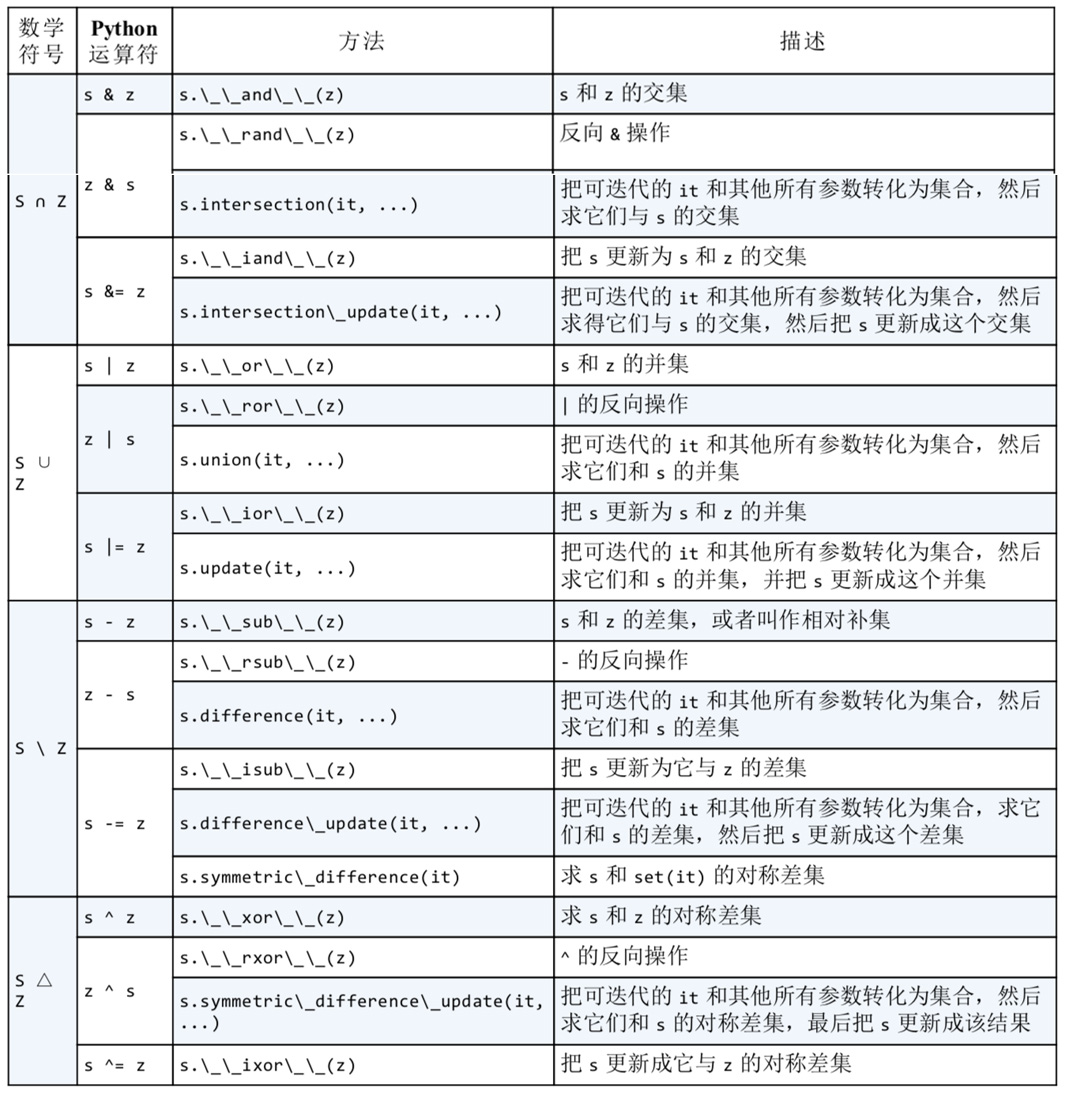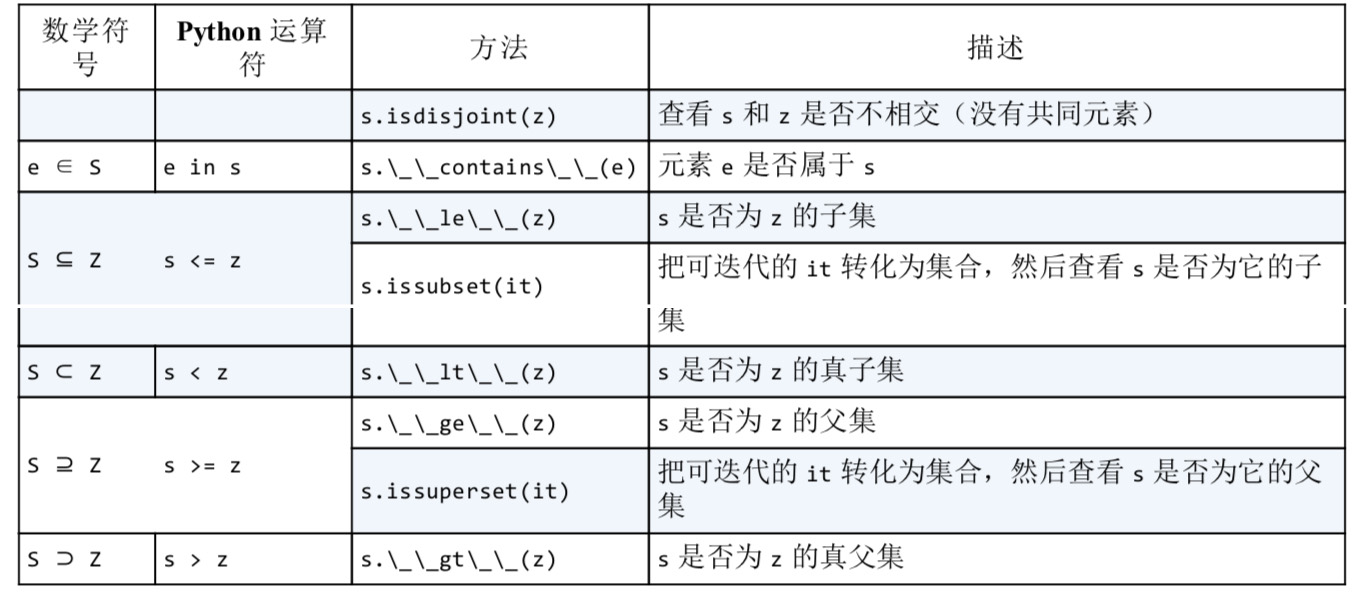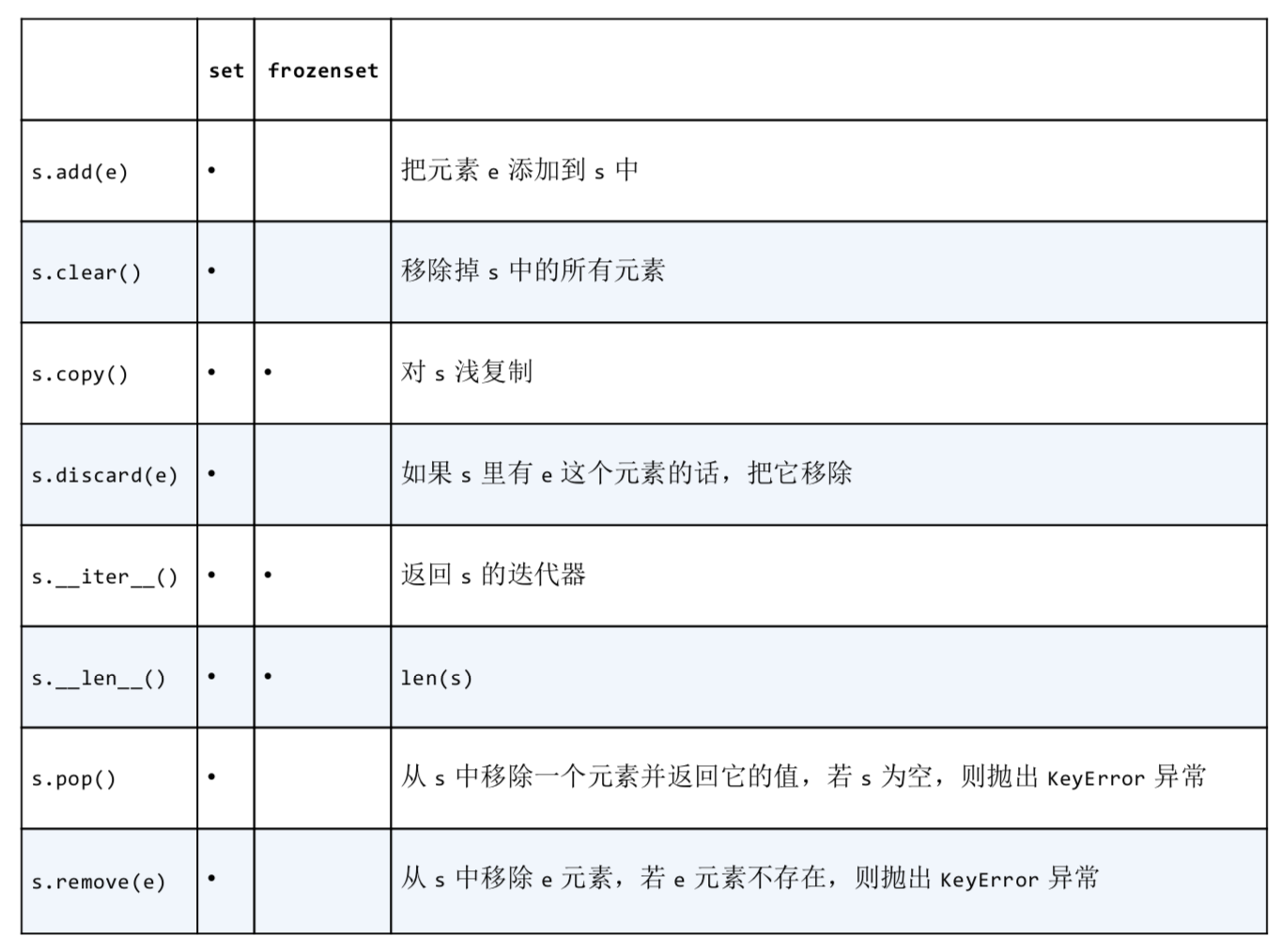### 可散列类型

 ``````''' 可散列数据类型（也称可hash）————我理解"可散列"就是"可hash" 可hash的对象需要实现__hash__方法，返回hash值；另外为了与其他对象比较还需要有__eq__方法 原子不可变数据类型（str、bytes和数值类型）都是可散列的，可散列对象必须满足下列要求： （1）实现了__hash__方法，并且所得到的hash值是不变的 （2）实现了__eq__方法，用来比较 （3）若a == b 为真，那么hash(a) == hash(b)也是真 ''' # 创建类Foo，并实现__hash__和__eq__ class Foo: def __init__(self, name): self.name = name def __hash__(self): print("正在hash...") return hash(self.name) def __eq__(self, other): print("正在比较...") return self.name == other.name def __repr__(self): return self.name if __name__ == "__main__": f1 = Foo("小李") f2 = Foo("小红") f3 = Foo("小李") s = set([f1, f2, f3]) # 集合实现不重复的原理正好利用了散列表 print(s) # {小红, 小李} print( f1 == f3, hash(f1) == hash(f3)) # True True 满足可散列对象的第三个条件``````
 ``````''' 对于元组来说，只有当一个元组包含的所有元素都是可hash的情况下，它才是可hash的 ''' t1 = (1, 2, 3, [1, 2]) # 元组里的列表的值是可变的，所以不可hash try: print(hash(t1)) except Exception as e: print(e) # unhashable type: 'list' t2 = (1, 2, 3, (1, 2)) # 元组里的元素都是不可变的，并且第二层元组里面的元素也不可变，所以可hash print(hash(t2)) # 3896079550788208169 t3 = (1, 2, 3, frozenset([1, 2])) print(hash(t3)) # -5691000848003037416``````

### 可散列类型

 ``````''' 可散列数据类型（也称可hash）————我理解"可散列"就是"可hash" 可hash的对象需要实现__hash__方法，返回hash值；另外为了与其他对象比较还需要有__eq__方法 原子不可变数据类型（str、bytes和数值类型）都是可散列的，可散列对象必须满足下列要求： （1）实现了__hash__方法，并且所得到的hash值是不变的 （2）实现了__eq__方法，用来比较 （3）若a == b 为真，那么hash(a) == hash(b)也是真 ''' # 创建类Foo，并实现__hash__和__eq__ class Foo: def __init__(self, name): self.name = name def __hash__(self): print("正在hash...") return hash(self.name) def __eq__(self, other): print("正在比较...") return self.name == other.name def __repr__(self): return self.name if __name__ == "__main__": f1 = Foo("小李") f2 = Foo("小红") f3 = Foo("小李") s = set([f1, f2, f3]) # 集合实现不重复的原理正好利用了散列表 print(s) # {小红, 小李} print( f1 == f3, hash(f1) == hash(f3)) # True True 满足可散列对象的第三个条件``````
 ``````''' 对于元组来说，只有当一个元组包含的所有元素都是可hash的情况下，它才是可hash的 ''' t1 = (1, 2, 3, [1, 2]) # 元组里的列表的值是可变的，所以不可hash try: print(hash(t1)) except Exception as e: print(e) # unhashable type: 'list' t2 = (1, 2, 3, (1, 2)) # 元组里的元素都是不可变的，并且第二层元组里面的元素也不可变，所以可hash print(hash(t2)) # 3896079550788208169 t3 = (1, 2, 3, frozenset([1, 2])) print(hash(t3)) # -5691000848003037416``````

``````my_dict.setdefault(key, []).append(new_value)
# 等同于
if key not in my_dict:
my_dict[key] = []
my_dict[key].append(new_value)
``````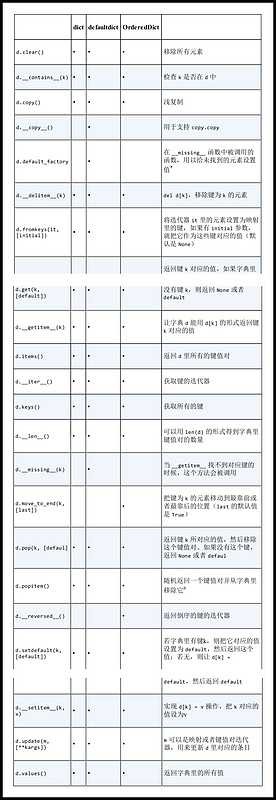``````>>> from types import MappingProxy Type
>>> d = {1: 'A'}
>>> d_proxy = MappingProxyType(d)
>>> d_proxy
mappingproxy({1: 'A'})
>>> d_proxy
'A'
>>> d_proxy = 'x'
Traceback(most recent call last):
File "<stdin", line 1, in <module>
TypeError: 'MappingProxy' object does not support item assignment
>>> d = 'B'
>>> d_proxy # d_proxy 是动态的，d 的改动会反馈到它上边
'B'
``````
``````>>> a = dict(one=1, two=2, three=3)
>>> b = {'one': 1, 'two': 2, 'three': 3}
>>> c = dict(zip(['one', 'two', 'three'], [1, 2, 3]))
>>> d = dict([('two', 2), ('one', 1), ('three', 3)])
>>> e = dict({'three': 3, 'one': 1, 'two': 2})
>>> a == b == c == d == e
True
``````

python 会设法保证大概有1/3 的表元是空的，所以在快要达到这个阈值的时候，原有的散列表会被复制到一个更大的空间。

Python内置的 hash() 方法可以用于计算所有的内置类型对象。

collections.OrderedDict collections.ChainMap collections.Counter 不可变的映射类型

`__missing__` 方法只会被 `__getitem__` 调用。提供 missing 方法对 get 或者 __contains__(in 运算符会用到这个方法)这些方法的是有没有影响。

contains 方法存在是为了保持一致性，因为 k in d 这个操作会调用它，但我们从 dict 继承到的 contains 方法不会在找不到键的时候用 missing 方法。

#### 您可能感兴趣的文章:

• Python数据结构与算法之常见的分配排序法示例【桶排序与基数排序】
• Python数据结构与算法之图的广度优先与深度优先搜索算法示例
• Python数据结构与算法之使用队列解决小猫钓鱼问题
• Python数据结构之栈、队列的实现代码分享
• Python数据结构之顺序表的实现代码示例
• Python数据结构与算法之链表定义与用法实例详解【单链表、循环链表】
• Python中顺序表的实现简单代码分享

Mapping 中提供了 get 方法，和我们在 StrKeyDict0 中定义的一样，所以我们在这里不需要定义 get 方法。

``````import collections

class StrKeyDict(collections.UserDict):

def __missing__(self, key):
if isinstance(key, str):
raise KeyError(key)
return self[str(key)]

def __contains__(self, key):
# 这里可以放心假设所有已经存储的键都是字符串。因此只要在 self.data 上查询就好了
return str(key) in self.data

def __setitem__(self, key, item):
# 这个方法会把所有的键都转化成字符串。
self.data[str(key)] = item
``````

collections.abc 模块中有 Mapping 和 MutableMapping 这两个抽象基类，它们的作用是为 dict 和其他类似的类型定义形式接口。

5、往字典里添加新键可能会改变已有键的顺序

defaultdict：处理找不到的键的一个选择

``````class StrKeyDict0(dict): # 继承 dict
def __missing__(self, key):
if isinstance(key, str):
# 如果找不到的键本身就是字符串，抛出 KeyError
raise KeyError(key)
# 如果找不到的键不是字符串，转化为字符串再找一次
return self[str(key)]
def get(self, key, default=None):
# get 方法把查找工作用 self[key] 的形式委托给 __getitem__，这样在宣布查找失败钱，还能通过 __missing__ 再给键一个机会
try:
return self[key]
except KeyError:
# 如果抛出 KeyError 说明 __missing__ 也失败了，于是返回 default
return default
def __contains__(self, key):
# 先按传入的键查找，如果没有再把键转为字符串再找一次
return key in self.keys() or str(key) in self.keys()
``````

2、字典开销巨大

``````import collections

index = collections.defaultdict(list)
index[new_key].append(new_value)
``````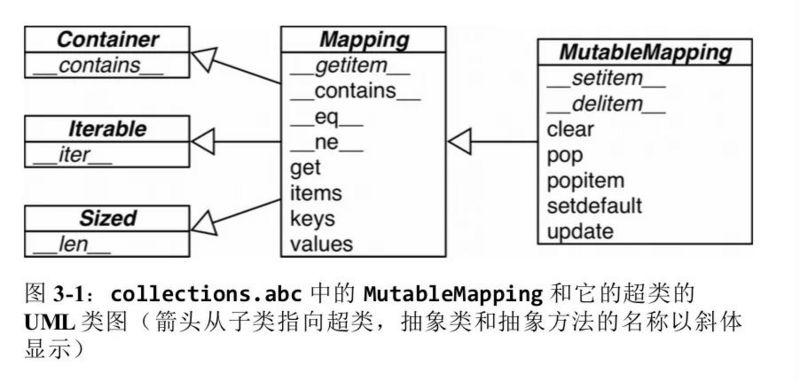1、键必须是可散列的

3、键查询很快

my_dict.keys() 在 Python3 中返回值是一个 "视图","视图"就像是一个集合，而且和字典一样速度很快。但在 Python2中，my_dict.keys() 返回的是一个列表。 所以 k in my_dict.keys() 操作在 python3中速度很快，但在 python2 中，处理效率并不高。4、键的次序决定于添加顺序

dict 的实现是典型的空间换时间：字典类型由着巨大的内存开销，但提供了无视数据量大小的快速访问。

TAG标签： 星彩网app下载
Ctrl+D 将本页面保存为书签，全面了解最新资讯，方便快捷。